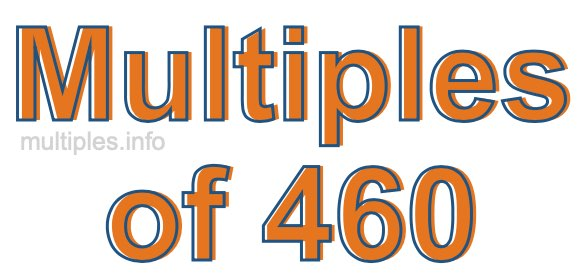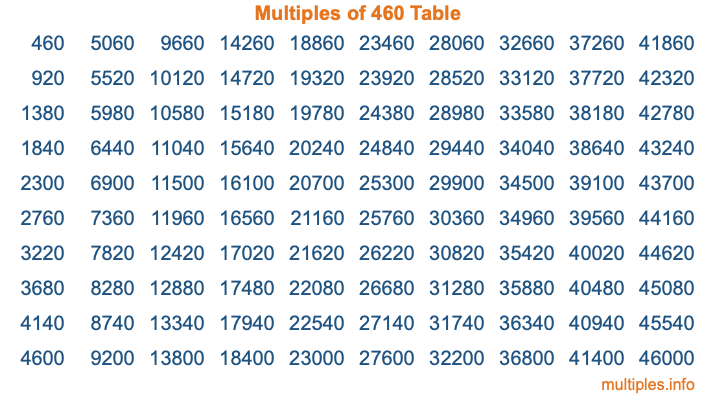Multiples of 460Welcome to the Multiples of 460 page. Here we will first teach you everything you will ever need to know about the multiples of 460, and then give you a study guide summary of everything we taught you to make sure you remember it all. Use this page to look up facts and learn information about the multiples of 460. This page will make you a multiples of four hundred sixty expert!

Definition of Multiples of 460
Multiples of 460 are all the numbers that when divided by 460 equal an integer. Each of the multiples of 460 are called a multiple. A multiple of 460 is created by multiplying 460 by an integer.

Therefore, to create a list of multiples of 460, you start with 1 multiplied by 460, then 2 multiplied by 460, then 3 multiplied by 460, and so on for as long as you want. Thus, the list of the first five multiples of 460 is 460, 920, 1380, 1840, and 2300. To see a larger list of multiples of 460, see the printable image of Multiples of 460 further down on this page. We also have a category where you can choose any nth multiple of 460.

Multiples of 460 Checker
The Multiples of 460 Checker below checks to see if any number of your choice is a multiple of 460. In other words, it checks to see if there is any number (integer) that when multiplied by 460 will equal your number. To do that, we divide your number by 460. If the the quotient is an integer, then your number is a multiple of 460.

Is  a multiple of 460?

Least Common Multiple of 460 and ...
A Least Common Multiple (LCM) is the lowest multiple that two or more numbers have in common. This is also called the smallest common multiple or lowest common multiple and is useful to know when you are adding our subtracting fractions. Enter one or more numbers below (460 is already entered) to find the LCM.

Check out our LCM Calculator if you need more details about the Least Common Multiple or if you need the LCM for different numbers for adding and subtraction fractions.

nth Multiple of 460
As we stated above, 460 is the first multiple of 460, 920 is the second multiple of 460, 1380 is the third multiple of 460, and so on. Enter a number below to find the nth multiple of 460.

th multiple of 460

Multiples of 460 vs Factors of 460
460 is a multiple of 460 and a factor of 460, but that is where the similarities end. All postive multiples of 460 are 460 or greater than 460. All positive factors of 460 are 460 or less than 460.

Below is the beginning list of multiples of 460 and the factors of 460 so you can compare:

Multiples of 460: 460, 920, 1380, 1840, 2300, etc.

Factors of 460: 1, 2, 4, 5, 10, 20, 23, 46, 92, 115, 230, 460

As you can see, the multiples of 460 are all the numbers that you can divide by 460 to get a whole number. The factors of 460, on the other hand, are all the whole numbers that you can multiply by another whole number to get 460.

It's also interesting to note that if a number (x) is a factor of 460, then 460 will also be a multiple of that number (x).

Multiples of 460 vs Divisors of 460
The divisors of 460 are all the integers that 460 can be divided by evenly. Below is a list of the divisors of 460.

Divisors of 460: 1, 2, 4, 5, 10, 20, 23, 46, 92, 115, 230, 460

The interesting thing to note here is that if you take any multiple of 460 and divide it by a divisor of 460, you will see that the quotient is an integer.

Multiples of 460 Table
Below is an image of the first 100 multiples of 460 in a table. The table is in chronological order, column by column. The first column has the first ten multiples of 460, the second column has the next ten multiples of 460, and so on.The Multiples of 460 Table is also referred to as the 460 Times Table or Times Table of 460. You are welcome to print out our table for your studies.

Negative Multiples of 460
Although not often discussed or needed in math, it is worth mentioning that you can make a list of negative multiples of 460 by multiplying 460 by -1, then by -2, then by -3, and so on, to get the following list of negative multiples of 460:

-460, -920, -1380, -1840, -2300, etc.

Multiples of 460 Summary
Below is a summary of important Multiples of 460 facts that we have discussed on this page. To retain the knowledge on this page, we recommend that you read through the summary and explain to yourself or a study partner why they hold true.

There are an infinite number of multiples of 460.

A multiple of 460 divided by 460 will equal a whole number.

460 divided by a factor of 460 equals a divisor of 460.

The nth multiple of 460 is n times 460.

The largest factor of 460 is equal to the first positive multiple of 460.

460 is a multiple of every factor of 460.

460 is a multiple of 460.

A multiple of 460 divided by a divisor of 460 equals an integer.

460 divided by a divisor of 460 equals a factor of 460.

Any integer times 460 will equal a multiple of 460.

Multiples of a Number
Here you can get the multiples of another number, all with the same attention to detail as we did for multiples of 460 on this page.

Multiples of
Multiples of 461
Did you find our page about multiples of four hundred sixty educational? Do you want more knowledge? Check out the multiples of the next number on our list!